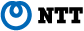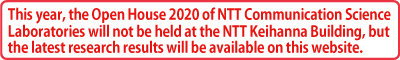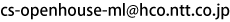Exhibition Program

Science of Machine Learning

05

Anomaly detection with low false positive rate

Semi-supervised learning for maximizing partial AUCAbstract

The partial area under a receiver operating characteristic curve (pAUC) is a performance measurement for binary classification problems that summarizes the true positive rate with the specific range of the false positive rate. Obtaining classifiers that achieve high pAUC is important in a wide variety of applications, such as anomaly detection and medical diagnosis. Although many methods have been proposed for maximizing the pAUC, existing methods require many labeled data for training. We propose a semi-supervised learning method for maximizing the pAUC, which trains a classifier with a small amount of labeled data and a large amount of unlabeled data. To exploit the unlabeled data, we derive two approximations of the pAUC: the first is calculated from positive and unlabeled data, and the second is calculated from negative and unlabeled data. A classifier is trained by maximizing the weighted sum of the two approximations of the pAUC and the pAUC that is calculated from positive and negative data.

References

1. T. Iwata, A. Fujino, N. Ueda, “Semi-supervised learning for maximizing the partial AUC,” in Proc AAAI Conference on Artificial Intelligence （AAAI）, 2020.

Contact

Tomoharu Iwata / Ueda Research Laboratory
Email: